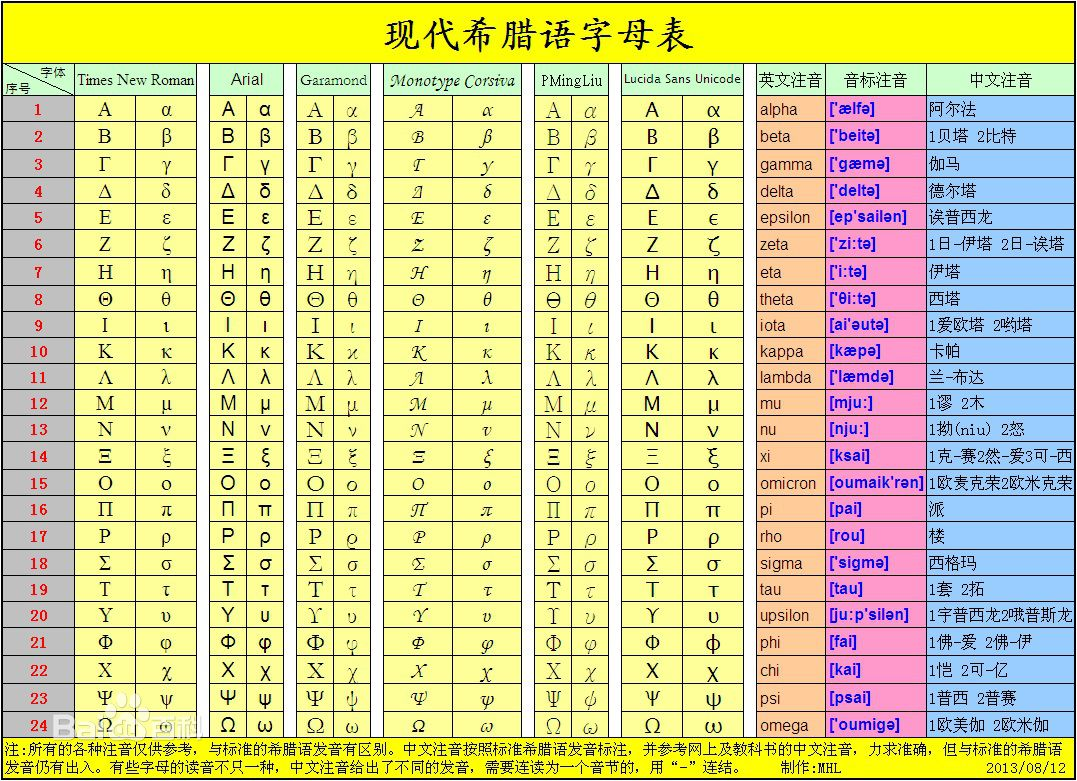# Java Lambda表达式使用及原理

## 前言

Lambda表达式使用了λ的英文名称。@FunctionalInterface
interface User {
void setName(String name);
}


User user = name -> System.out.println("Hello " + name);


1:变量长期驻扎在内存中；
2:避免全局变量的污染；
3:私有成员的存在 ；

• 由于闭包会使得函数中的变量都被保存在内存中，内存消耗很大，所以不能滥用闭包，否则会造成网页的性能问题，在IE中可能导致内存泄露。解决方法是，在退出函数之前，将不使用的局部变量全部删除。
• 闭包会在父函数外部，改变父函数内部变量的值。所以，如果你把父函数当作对象（object）使用，把闭包当作它的公用方法（Public Method），把内部变量当作它的私有属性（private value），这时一定要小心，不要随便改变父函数内部变量的值。

Lambda表达式分三部分：

• 入参：
• 箭头符号： —>
• 方法体：

## 基本使用

()=>Console.WriteLine("This is a Lambda expression.");

m=>m*2;   等效于   (m)=>m*2

(m,n)=>m*n;

(m,n)=>{int result=m*n; Console.WriteLine(result);}


https://www.runoob.com/java/java8-functional-interfaces.html

/**
* java8四大核心函数式接口
* Consumer<T> :消费接口
*      void accept(T t);
*
*
* Suppliter<T>:供给型接口
*      T get();
*
*
* Function<T,R>:函数型接口
*      R apply(T t);
*
*
* Predicate<T> : 断言型接口
*      boolean test(T t);
*/

------------------------------------------------------------
/**
* Consumer<T> :消费接口
*/
@Test
public void test1() {
happy( 100,(x) -> System.out.println("消费"+x));
}

public void happy(double d, Consumer<Double> consumer) {
consumer.accept( d );
}

------------------------------------------------------------
/**
*Suppliter<T>:供给型接口
*/
@Test
public void test2() {
List <Integer> i = getLsut(10,() -> (int)Math.random()*100);
i.forEach( (o) -> System.out.println(o));
}

/**
* 得到任意长度的集合
*/
public List<Integer> getLsut(int i, Supplier<Integer> supplier) {
List<Integer> list = new ArrayList <>(  );
for(int j = 0;i<=i;++j) {
Integer integer = supplier.get();
list.add( integer );
}
return list;
}

------------------------------------------------------------
/**
*Function<T,R>:函数型接口
*/
@Test
public void test3() {
getString( "sdfds  ",(x1) -> x1.trim() );
}

/**
* 去掉字符串中的空格
*/
public String getString(String s, Function<String,String> function) {
return function.apply( s );
}

------------------------------------------------------------
/**
*Predicate<T> : 断言型接口
*/
@Test
public void test4() {
List<String> sli = Arrays.asList( "dddd","fgg","gfjjg","fsdfsgdf" );
List<String> list = checkString(sli,(s) -> s.length()>5);
list.forEach( (s) -> System.out.println(s));
}

/**
* 将满足条件的数据放在集合中
*/
public List<String> checkString(List<String> list, Predicate<String> predicate) {
List<String> slist = new ArrayList <>(  );
list.forEach( (o) -> {
if(predicate.test( o )) {
slist.add( o );
}
} );
return slist;
}


Java8新特性

https://www.runoob.com/java/java8-new-features.html


## 原理

Lambda表达式

//定义表达式样例

@FunctionalInterface
interface Print<T> {
public void print(T x);
}

public class Lambda {
public static void PrintString(String s, Print<String> print) {
print.print(s);
}

public static void main(String[] args) {
PrintString("test", (x) -> System.out.println(x));
}
}


public class Lambda {
public static void PrintString(String s, Print<String> print) {
print.print(s);
}
private static void lambda$0(String x) { System.out.println(x); } final class$Lambda$1 implements Print{ @Override public void print(Object x) { lambda$0((String)x);
}
}
public static void main(String[] args) {
PrintString("test", new Lambda().new $Lambda$1());
}
}


## 总结

• lambda表达式：可以用来表示函数的语法糖，本质是一个匿名函数。
• 在类编译时，会生成一个私有静态方法+一个内部类；
• 在内部类中实现函数式接口，在实现接口的方法中，调用编译器生成的静态方法；
• 在使用lambda表达式的地方，通过传递内部类实例，来调用函数式接口方法。01-12159
08-071875
09-208050
04-061万+
07-191039
05-21214
12-133112
09-05650
02-1353
09-2088
02-2544
11-18363
08-01334
12-0313
02-071万+
02-2568
12-1075
©️2020 CSDN 皮肤主题: 大白 设计师:CSDN官方博客